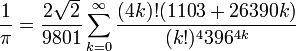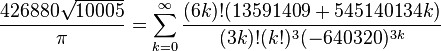Evaluating Pi …

… to 15 decimal places in one easy step (or not too difficult step).

In my previous post one of the examples given of the use of the Gaussian Quadrature function was to evaluate the value of Pi.  Let’s look at that a bit more closely.

Wikipedia has a nice article on the history of Pi, but like most such articles the methods described are either historical methods which are easy to understand but converge slowly, or recent methods which converge very quickly, but which are presented with little or no information about how they were derived.  For example the well known 20th Century Indian mathematician Srinivasa Ramanujan somehow derived the relationship:$\frac{1}{\pi} = \frac{2 \sqrt 2}{9801} \sum_{k=0}^\infty \frac{(4k)!(1103+26390k)}{(k!)^4 396^{4k}}\!$

and in 1987 the Chudnovsky brothers  derived:$\frac{426880 \sqrt{10005}}{\pi} = \sum_{k=0}^\infty \frac{(6k)! (13591409 + 545140134k)}{(3k)!(k!)^3 (-640320)^{3k}}\!$

which delivers 14 digits per term, but no indication is given of how these relationships were derived.

The early historical methods of calculating Pi, starting with Archimedes in the 3rd century BC, were based on the successive subdivision of polygons.Around AD 265, the Wei Kingdom mathematician Liu Hui provided a simple and rigorous iterative algorithmto calculate π to any degree of accuracy. He himself carried through the calculation to a 3072-gon and obtained an approximate value for π of 3.1416.A simple way to improve the accuracy of the polygon approach is to find an approximation of the area between the circle and the polygon, and add this to the known area of the polygon.  Starting with a hexagon of unit side, this may be divided into 6 equilateral triangles and six circular segments with 60 degree included angle:

The area of the triangle is 0.5 x 3^0.5 / 2 (0.5 x base x height)

The equation of the segment is (1 – x^2)^0.5 – 3^0.5 / 2

If this equation is integrated between x = -0.5 and x = 0.5, and added to the area of the equilateral triangle, this gives 1/6 the area of the circle of unit radius.  If the integration is evaluated using Gaussian Quadrature, with 8 integration points, the resulting area provides an estimate  of Pi with an error of 2.3E-11; not too bad:

A further 4 significant figures can be obtained by simply changing the limits of integration to X = 0 to 0.5 (and adjusting the triangle area to suit, and multiplying the resulting area by 12):

The resulting estimate of Pi has an error of 7.6E-15, and matches the built in Excel Pi function to the 14th significant figure.

This entry was posted in Excel, Maths, Newton, UDFs, VBA and tagged , , , , . Bookmark the permalink.

4 Responses to Evaluating Pi …

1.paviavio says:

This would be very good piece for Pi Day.

Like

2.dougaj4 says:

“This would be very good piece for Pi Day.”

I didn’t think of that.

Pity there aren’t 31 days in April.

I’ll just have to wait for 22/July 🙂

Like

3.خرید کریو says:

thank you

Like

This site uses Akismet to reduce spam. Learn how your comment data is processed.